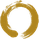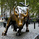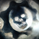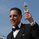3420 views
3420
The Linear Regression Slope is based on the Curve.

List of All my Indicators - https://www.tradingview.com/p/stocks/?so...
```// Created by UCSgears -- Version 1
study(title="UCSGEARS - Linear Regression Curve", shorttitle="UCS-LRC", overlay=true)
src = close
len = input(defval=25, minval=1, title="Linear Regression Length")
lrc = linreg(src, len, 0)
plot(lrc, color = red, title = "Linear Regression Curve", style = line, linewidth = 2)```Hi,

I'm trying to code a Linear Regression Band indicator that has the same standard deviations as the built in Linear Regression indicator/drawing tool.

The mean of my indicator (code below) is correct but the standard deviation bands are always in the wrong place when I compare them to the same length LR indicator or drawing tool.

Does anyone know how to fix this issue so that the bands match the built in drawing tool/indicator?

Here is my code ...

//@version=3
study(shorttitle="LRB", title="Linear Regression Bands", overlay=true)
source = close
length = input(50, minval=1)
offset = 0
mult = input(2, title="StDev", minval=0.001, maxval=5)
basis = linreg(source, length, offset)
dev = mult * stdev(source, length)
upper = basis + dev
lower = basis - dev
plot(basis, color=red)
plot(upper, color=blue)
plot(lower, color=blue)

Many thanks! PeteIs there a way to put standard deviations onto this indicator so it is a moving linear regression channel?Yes, Use the Bollinger Band code and replace the SMA/EMA with the LRC.Thanks for a great work as usual, USC!

I would like to ask you for a small improvement to this one: the ability to apply the price calculations of the slope to High-Low-Open-Close prices.

I also need the ability to do the same in a DEMA (Double Exp MA) if you can help me :)